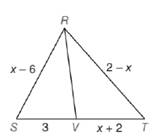Chapter 5.6, Problem 25EElementary Geometry For College St...

7th Edition
Alexander + 2 others
ISBN: 9781337614085

Solutions

Chapter
SectionElementary Geometry For College St...

7th Edition
Alexander + 2 others
ISBN: 9781337614085
Textbook Problem

Given: R V → bisects ∠ S R T , R S = x − 6 , S V = 3 , R T = 2 − x , and V T = x + 2Find: x (HINT: You will need to apply the Quadratic Formula.)

To determine

To find:

The value of x.

Explanation

Given:

RV bisects SRT, RS=x6,SV=3,RT=2x,VT=x+2.

Theorem used:

Angle bisector theorem:

If a ray bisects one angle of a triangle, then it divides the opposite side into segments whose lengths are proportional to the lengths of the two sides that form the bisected angle.

Calculation:

We have RV bisects SRT.

By angle bisector theorem, we get

SRRT=SVVT.

Given that RS=x6,SV=3,RT=2x,VT=x+2

Thus x62x=3x+2.

Now let us find x.

x62x=3x+2(x6)(x+2)=3(2x)x2+2x6x12=63xx24x126+3x=0x2x18=0

Let us find the value of x using the quadratic formula, x=b±b24ac2a

Still sussing out bartleby?

Check out a sample textbook solution.

See a sample solution

The Solution to Your Study Problems

Bartleby provides explanations to thousands of textbook problems written by our experts, many with advanced degrees!

Get Started

Prove that limx21x=12.

Calculus (MindTap Course List)

In problems 1-6, perform the indicated matrix operations with the following matrices.

Mathematical Applications for the Management, Life, and Social Sciences

Differentiate. f(x) = (3x2 5x)ex

Single Variable Calculus: Early Transcendentals

Solve for x: e2x1 = 10. a) 12(1+10e) b) 3 + ln 10 c) 2 + ln 10 d) 1+ln102

Study Guide for Stewart's Single Variable Calculus: Early Transcendentals, 8th